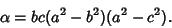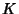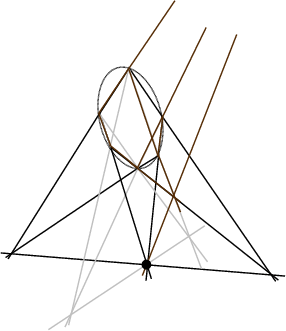## Steiner Points

There are two different types of points known as Steiner points.

The point of Concurrence of the three lines drawn through the Vertices of a Triangle Parallel to the corresponding sides of the first Brocard Triangle. It lies on the Circumcircle opposite the Tarry Point and has Triangle Center FunctionThe Brianchon Point for Kiepert's Parabola is the Steiner point. The Lemoine Pointis the Steiner point of the first Brocard Triangle. The Simson Line of the Steiner point is Parallel to the line, whenis the Circumcenter andis the Lemoine Point.If triplets of opposites sides on a Conic Section in Pascal's Theorem are extended for all permutations of Vertices, 60 Pascal Lines are produced. The 20 points of their 3 by 3 intersections are called Steiner points.

See also Brianchon Point, Brocard Triangles, Circumcircle, Conic Section, Kiepert's Parabola, Lemoine Point, Pascal Line, Pascal's Theorem, Steiner Set, Steiner Triple System, Tarry Point

References

Casey, J. A Treatise on the Analytical Geometry of the Point, Line, Circle, and Conic Sections, Containing an Account of Its Most Recent Extensions, with Numerous Examples, 2nd ed., rev. enl. Dublin: Hodges, Figgis, & Co., p. 66, 1893.

Gallatly, W. The Modern Geometry of the Triangle, 2nd ed. London: Hodgson, p. 102, 1913.

Johnson, R. A. Modern Geometry: An Elementary Treatise on the Geometry of the Triangle and the Circle. Boston, MA: Houghton Mifflin, pp. 281-282, 1929.

Kimberling, C. Central Points and Central Lines in the Plane of a Triangle.'' Math. Mag. 67, 163-187, 1994.